﻿ Global Analysis of Rectifying Antenna with GaN Schottky Barrier Diode using WCIP Method for Wireless Power Transmission

### Global Analysis of Rectifying Antenna with GaN Schottky Barrier Diode using WCIP Method for Wireless...

Zied Harouni, Lassaad Latrach, Lotfi Osman, Ali Gharsallah, Henri BaudrandOPEN ACCESSPEER-REVIEWED

## Global Analysis of Rectifying Antenna with GaN Schottky Barrier Diode using WCIP Method for Wireless Power Transmission

Zied Harouni1,, Lassaad Latrach1, Lotfi Osman1, Ali Gharsallah1, Henri Baudrand2

1Laboratoire d’Electronique Département de physique, Faculté des Sciences de Tunis, El Manar, Tunisia

2Laboratoire d’Electronique ENSEEIHT de Toulouse France

### Abstract

A precise technique based on the wave concept iterative procedure (WCIP) and a fast mode transformation (FMT) is used to analyze a rectifying antenna (Rectenna) circuit. This global analysis is achieved by using surface impedance to model the nonlinear element (GaN Schottky Barrier diode). These systems use the manufacturing processes of integrated circuits. In order to initialize the iterative procedure, an incident wave is defined in spectral domain. The numerical results are compared to those obtained with the measured one. The good agreement between simulated and published data justifies the design procedure.

### At a glance: Figures

123
Prev Next

• Harouni, Zied, et al. "Global Analysis of Rectifying Antenna with GaN Schottky Barrier Diode using WCIP Method for Wireless Power Transmission." International Transaction of Electrical and Computer Engineers System 2.1 (2014): 14-18.
• Harouni, Z. , Latrach, L. , Osman, L. , Gharsallah, A. , & Baudrand, H. (2014). Global Analysis of Rectifying Antenna with GaN Schottky Barrier Diode using WCIP Method for Wireless Power Transmission. International Transaction of Electrical and Computer Engineers System, 2(1), 14-18.
• Harouni, Zied, Lassaad Latrach, Lotfi Osman, Ali Gharsallah, and Henri Baudrand. "Global Analysis of Rectifying Antenna with GaN Schottky Barrier Diode using WCIP Method for Wireless Power Transmission." International Transaction of Electrical and Computer Engineers System 2, no. 1 (2014): 14-18.

 Import into BibTeX Import into EndNote Import into RefMan Import into RefWorks

### 1. Introduction

Within the framework of the recovery of energy for wireless sensor networks, the energy-harvesting system using the rectenna has a big interest [1, 2, 3]. This paper presents a global analysis of (Rectenna) rectifying antenna by using surface impedance to model the nonlinear element (GaN Schottky Barrier diode). The Rectenna behaving like a wireless battery is a necessary device to convert the energy RF in direct current. Usually, a Rectenna consists of a microwave antenna to collect incoming RF power, an input low-pass filter (LPF) to suppress unwanted higher harmonics rejected by the rectifying circuit [4, 5, 6, 7], an output dc pass filter, and a resistive load. In Rectenna design, simulation is an essential step in modeling this type of circuit. In this framework, several analytical methods have been developed [5, 9]. Among these methods that already exist, we present in what follows a new iterative method based on the wave concept [9, 10, 11, 12, 13]. This method has major advantages over other methods. These advantages are concerning, in special, the execution velocity of the resolution procedure and the arbitrary form of the under studied structure. Besides this iterative technique uses a rapid transformation FMT which ensures a rapid transition between the spectral and spatial domain . We combine the wave concept with the two dimensions Fast Fourier Transformation (2D-FFT) algorithm to change the domain. The use of the 2D-FFT algorithm is required to mesh the circuit plane into 2D small rectangular pixels the 2D-FFT algorithm, a high computational speed can be achieved .

### 2. Theory

A brief overview of the WCIP method [3-8] based on wave concept is presented here. It consists of generating a recursive relationship between a given incident and reflected waves generated from the discontinuity planes (eq.1). The analysis structure shown in Figure 1 is composed of planar microstrip enclosed in the metal box; the interface Ω is divided into cells which form sub-domains corresponding to metal, dielectric and sources.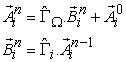(1)

The wave concept is introduced by writing the tangential electric field E, (on Ω) and surface tangential current density J, (on Ω) in terms of incident and reflected waves.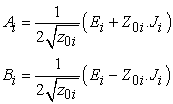(2)

where Ji is the surface tangential current density which is defined as: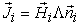; ni is a unit vector normal to Ω. Ai and Bi are two tangential vectors associated with the discontinuity interface Ω. Z0i is the characteristic impedance of region i (Eq. 3):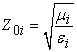(3)

εi, µi are the permittivity and permeability of region I, respectively. Ei and Hi are the tangential electric and magnetic field on Ω. The interface Ω is characterized by a scattering operation matrix depending on boundary conditions defined for each point of Ω. The continuity conditions for fields in each point of Ω (spatial domain) are:

E1 = E2 = 0 on the metal domain.

E1 = E2 and J1 + J2 = 0 on the dielectric domain.

E1 = E2 = E0 on the source domain.

In this section we focus on the study of planar microwave structures incorporating Schottky diodes  in order to provide specific functions. Figure 2 shows a localized element inserted into a planar circuit.

ZL is the impedance of equivalent circuit element, the electric field in the area of the element is connected to the voltage V by the equation: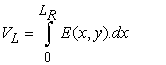(4)

The current density is related to the tangential current density by: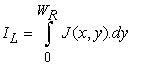(5)

The dimensions of the element are very small spot in front of the working wavelength λg, (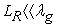) and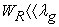), then we can assume that E and J are uniform in the area of the element.

Equations (4) and (5) become: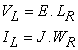Thus, we can define the surface impedance ZS by: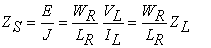(6)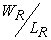is the form factor of the element located and ZL is the impedance of the equivalent circuit.

To study a circuit containing such an element by the iterative method which is based on the concept of waves, it is necessary to determine the relation between the incidental waves and the waves reflected in its domain, and to add it in the expression of the operator diffraction in the spatial domain.

Hz is the matrix on the area of the element, the boundary conditions in this area are: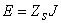.

In terms of waves, we can write: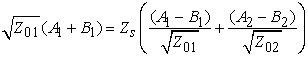(7)

The operator of this field diffraction is: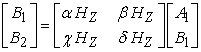(8)

with: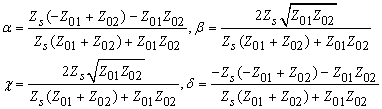The diffraction operator of the surface impedance in the diode region becomes: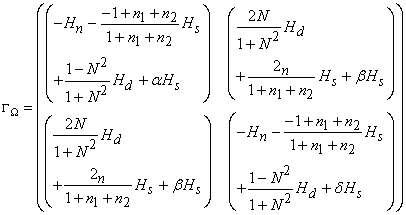The equivalent circuit of the circuit with the Schottky diode is shown in Figure 3.

Cp = 0.05 pF is a parasitic capacitance, Lp = 0.5 nH is a parasitic inductance, and Cj(V) is a variable capacitor: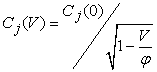where Cj(0) = 1pF is the junction capacitance at zero bias potential and is called the integrated (built-in potential). The boundary conditions in the area of the Schottky diode given by the equivalent circuit are: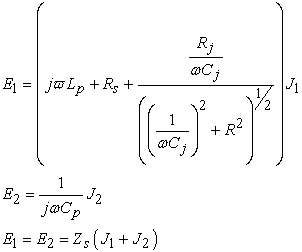or: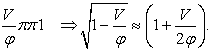For this diode, the form factor is 1. The operator for the diffraction field of the diode is: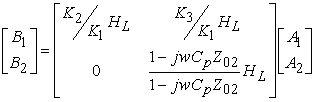with: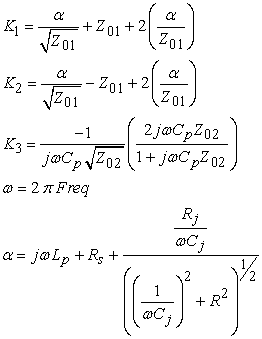### 3. Applications

We propose here an analysis of a Rectenna circuit at 2.45 GHz in microstrip technology previously studied by Alexander et al Douyère . It consists of a rectangular patch antenna with linear polarization and a conversion circuit series Schottky diode. The two parts of the Rectenna have been adapted on 50 Ω impedance. In addition, the supply line between the antenna and the converter circuit has a width W1 = and a characteristic impedance of 50 Ω. The Schottky diode has been rigorously modeled by an impedance surface and introduced into the calculation of the method.

To reconstruct the process of measuring a complete Rectenna illuminated by an incident plane wave inside an anechoic chamber, the global simulation based on the iterative method was used. The distributions of the electric field and surface current were calculated. They will be presented and used to demonstrate the performance of the Rectenna circuit in terms of performance and compactness. The results are routinely compared with measurements. In this case, the structure is excited by a plane wave at normal incidence with a polarization along (ox).

Figure 4 shows the Rectenna using a Schottky diode.

#### Table 1. Dimensions of the Rectenna in mmDownload asPowerPoint Slide

Veiw figureView current table in a new window

The circuit consists of a square patch antenna with linear polarization and a conversion circuit series. In addition, a matching circuit between the antenna and the converter circuit was designed. The Rectenna is printed on a teflon glass substrate (εr = 3.2, tan δ = 0.003 and h = 0762 mm). The number of iterations required for convergence of results is obtained from 200 iterations. The total structure is synthesized using a mesh of 128 × 128 pixels with basic dimensions of Δx = 0.71 mm and Δy = 0.77 mm. Figure 5 shows the discretization of the circuit plane.

The space contains two main sub-areas, namely the space containing metal, dielectric, and load and frequency domain that contains the source area of the incident plane wave form. We need to determine the diffraction operator in each area based on the boundary conditions for each area. The mesh size of space is done by dividing the interface containing the circuit in rectangular pixels, so we can define a matrix on each area. (Hm: metalworking, Hd: dielectric and field Hs: field of the diode and Hc: domain of the load) that takes the value the pixels that represent the field and 0 elsewhere.

The 3D representations of electric fields (Figure 6) and current densities (Figure 7) to 2.45 GHz frequency obtained after a computation time of 1 min 05 s show that the boundary conditions are met at every point of the interface containing the circuit since the sum of current densities is zero on the dielectric and density fields is zero on the metal.

For an electrical device (microwave-RF-low frequency or other) to be usable, it must present a stable condition during its simulation. Thus, by studying the convergence of the admittance seen by the source (Yin) versus number of iterations, we can conclude that the electrical stability is obtained.

The curve in Figure 8 shows that the convergence of the admittance seen by the source (Yin) is reached fairly quickly, in less than 200 iterations.

The analysis concludes by comparing simulation results with measurement results. From the curves shown in Figure 9, the maximum efficiency of the Rectenna is obtained for a power of 15 dBm and a load of 200 Ω. The simulation results obtained with the iterative method are compared with measured results from reference .

The efficiency of the Rectenna raised to power densities ranging from 0 to 35 mW is plotted in Figure 9. We note that there is good agreement between the two gaits. The good consistency between WCIP and results from any action is undeniable.

### 4. Conclusion

We have used in this paper a method to determine an overall characterization of an energy recovery circuit (Rectenna) incorporating an active non-linear component (Schottky diode). The iterative method adopted could be applied to model the diode by an impedance surface. A Matlab program was developed for the simulation of the method. The comparison of our results with those available in the literature validates our approach.

### References

  Ismail, M.F, Rahim, M.K.A, Hamid, M.R, Majid, H.A, Yusof, M.F.M. “Compact dual-fed slotted circular polarization antenna with reflector for RF energy harvesting, Antennas and Propagation (Eu CAP), 2013 7th European Conference on, 2013, Page(s): 2096-2099.In article  Franciscatto, Bruno R; Freitas, Vitor ; Duchamp, Jean-Marc; Defay, Christian; Vuong, Tan Phu, “High-efficiency rectifier circuit at 2.45 GHz for low-input-power RF energy harvesting’ Microwave Conference (Eu MC), 2013 European, 2013, Page(s): 507-510.In article  Szarka, G.D.; Burrow, S.G.; Proynov, P.P.; Stark, B.H., Power Electronics, IEEE Transactions on Volume: 29, Issue: 1, 2014, Page(s): 201-212In article  Y. H. Suh, C. Wang, and K. Chang, “Circularly polarized truncated corner square patch microstrip rectenna for wireless power transmission,” Electron. Let, vol. 36, no. 7, pp. 600-602, Mar. 2000.In article CrossRef  H. Takhedmit, B. Merabet, L. Cirio, B. Allard, F. Costa, C. Vollaire and O. Picon, “Analyse et conception de rectennas à 2.45 Vollaire and O. Picon, “Analyse et conception de rectennas à 2.45 GHz par une technique d’analyse globale”, Journées Nationales Micro-ondes, JNM 2009, 27-29, Mai 2009, Grenoble.In article  Harouni, Z.; Cirio, L.; Osman, L.; Gharsallah, A.; Picon, O, “A Dual Circularly Polarized 2.45-GHz Rectenna for Wireless Power Transmission,” Antennas and Wireless Propagation Letters, IEEE , Vol. 10, pp. 306-309, April 2011.In article CrossRef  Zied Harouni, Lotfi Osman, Laurent Cirio, Ali Gharsallah, Odile Picon, “Design Analysis of Rectenna for 2.45 GHz Wireless Power Transmission,” 6th, International Conference on Electrical Systems and Automatic Control. 26-28 Mars 2010, Hammamet, Tunisie.In article  A.Gharsallah, A. Gharbi and H. Baudrand, “Efficient analysis of multiport passive circuits using iterative technique,” Electromagnetics, vol. 21, 2001, pp. 73-84.In article CrossRef  N. Sboui, “Contribution à l’étude des circuits planaires par une méthode itérative,’’ Thèse de doctorat, Faculté des Sciences de Tunis, Tunisie, 2002.In article  H. Baudrand, R.S. N’gongo, “Applications of wave concept iterative procedure,” Recent RE. Devel. Microwave an Tech vol 1, pp 187-197, 1999.In article  N. Sboui, A. Gharsallah, H. Baudrand, A. Gharbi, “Global Modeling of Microwave Active Circuits by an Efficient Iterative Procedure,” IEE Proc.-Microw. Antennas Propag, Vol. 148, No. 3. June 2001.In article CrossRef  Latrach, L., N. Sboui, A. Gharsallah, A. Gharbi, and H. Baudrand, “Analysis and design of a planar multilayered FSS with arbitrary incidence,” Applied Computational Electromagnetics SocietyJournal, Vol. 23, No. 2, 149-154, Jun. 2008.In article  L. Latrach, N. Sboui, A. Gharsallah, and A. Gharbi, “A design and modelling of microwave active screen using a combination of the rectangular and periodic waveguides modes,” J. of Electromagn. Waves and Appl., Vol. 23, 1639-1648, 2009.In article  Kazimirchik, V. Nelayev, V. Sjakerskii, V, “Simulation of Schottky diode technology and performances for RFID application,” CAD Systems in Microelectronics, 2009. CADSM 2009. 10th International Conference-The Experience of Designing and Application, 2009, Page(s): 37-38.In article  Douyére, J.D. Lan Sun Luk and F. Alicalapa, “High efficiency microwave rectenna circuit: modelling and design,” Elect. lett. Vol. 44, No. 24, pp 1409-1410, November 2008.In article